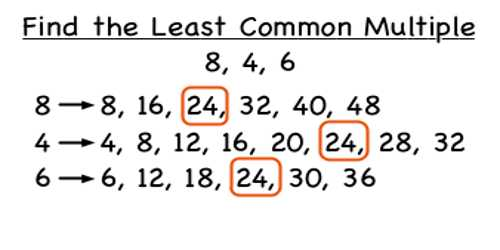Least Common Multiple

The Least Common Multiple (LCM) is the smallest number that two or more numbers will divide into evenly. The LCM of two numbers is the smallest number (not zero) that is a multiple of both.

How to find the Least Common Multiple of two numbers:

• Find the Greatest Common Factor (GCF) of the numbers
• Multiply the numbers together
• Divide the product of the numbers by the GCF.

Example: Find the LCM of 15 and 12

• Determine the Greatest Common Factor of 15 and 12 which is 3
• Either multiply the numbers and divide by the GCF (15*12=180, 180/3=60)
• OR – Divide one of the numbers by the GCF and multiply the answer times the other number (15/3=5, 5*12=60)Explanation

The least common multiple, or LCM, is another number that’s useful in solving many math problems. Let’s find the LCM of 30 and 45. One way to find the least common multiple of two numbers is to first list the prime factors of each number

30 = 2 × 3 × 5

45 = 3 × 3 × 5

Then multiply each factor the greatest number of times it occurs in either number. If the same factor occurs more than once in both numbers, you multiply the factor the greatest number of times it occurs.

2: one occurrence

3: two occurrences

5: one occurrence

2 × 3 × 3 × 5 = 90 ←LCM

After you’ve calculated a least common multiple, always check to be sure your answer can be divided evenly into both numbers.

EXAMPLES

Find the LCM of these sets of numbers.

3, 9, 21

Solution: List the prime factors of each.

3: 3

9: 3 × 3

21: 3 × 7

Multiply each factor the greatest number of times it occurs in any of the numbers. 9 has two 3s, and 21 has one 7, so we multiply 3 two times, and 7 once. This gives us 63, the smallest number that can be divided evenly by 3, 9, and 21. We check our work by verifying that 63 can be divided evenly by 3, 9, and 21.

12, 80

Solution: List the prime factors of each.

12: 2 × 2 × 3

80: 2 × 2 × 2 × 2 × 5 = 80

Multiply each factor the greatest number of times it occurs in either number. 12 has one 3, and 80 has four 2’s and one 5, so we multiply 2 four times, 3 once, and five once. This gives us 240, the smallest number that can be divided by both 12 and 80. We check our work by verifying that 240 can be divided into both 12 and 80.

Information Source;